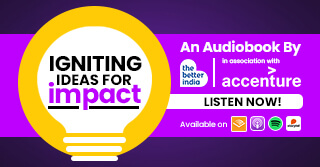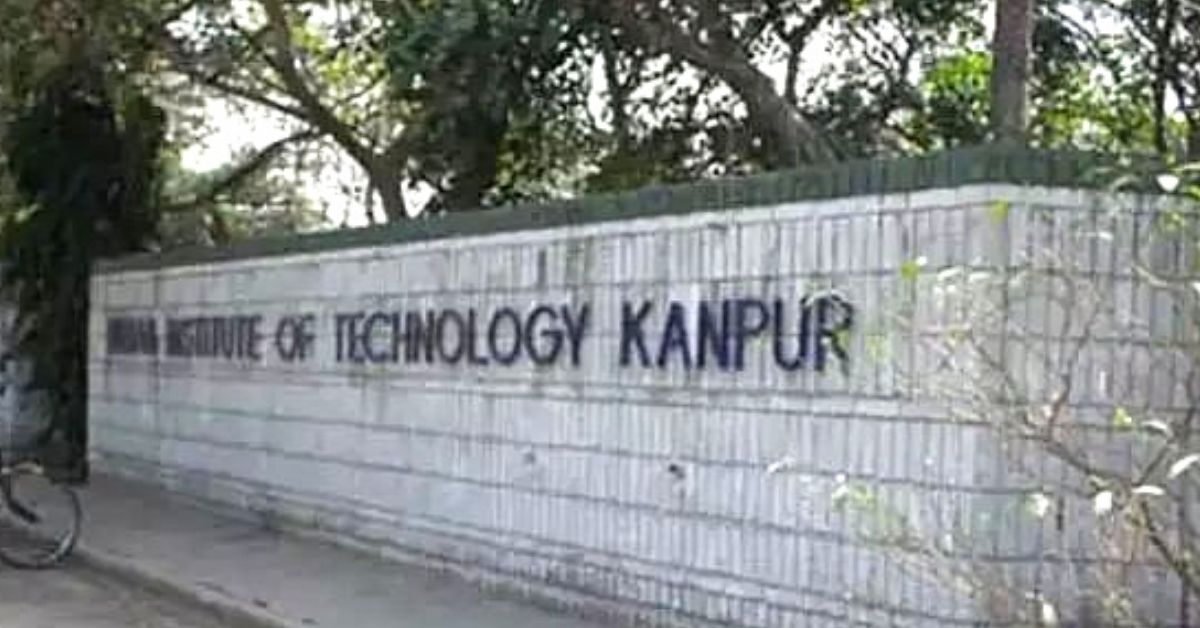Embarking on a transformative journey through six chapters, we traverse India's landscape, exploring pioneering startups and their revolutionary...

2 months

# IIT Kanpur Offers 2 Free Online Courses on Data Science, With CertificatesLearn the essentials of data software for free at IIT Kanpur. Read on for registration details, how to apply and more.

IIT Kanpur is offering two new free online courses on data science.
Essentials of Data Science With R Software – 1: Probability and Statistical Inference and Essentials of Data Science With R Software – 2: Sampling Theory and Linear Regression Analysis are the two courses being offered.

## Things to know:

• This is a two-part course being offered by IIT Kanpur.
• The course is absolutely free of charge.
• The course is being conducted by Dr Shalabh, who is a Professor of Statistics at the Indian Institute of Technology Kanpur.
• His research areas of interest are linear models, regression analysis and econometrics, and the professor has conducted several workshops on statistics for teachers, researchers and practitioners.
• Both courses offered by IIT Kanpur will be for a duration of 12 weeks.
• Those candidates who wish to appear for the examination and receive a certificate will have to pay Rs 1000.
• Only e-certificates will be dispatched. No hard copy will be sent out.

## What will you learn?

Part 1

Candidates will get to learn the following modules: Introduction to data science, basic calculations with R Software and probability theory, probability theory and random variables, random variables and discrete probability distributions, continuous probability distributions, sampling distributions and functions of random variables, convergence of random variables, central limit theorems and law of large numbers, statistical inference and point estimation, methods of point estimation of parameters, point and confidence interval estimation, confidence interval estimation and test of hypothesis, test of hypothesis, and test of hypothesis for attributes and other tests.

Part 2

Candidates will get to learn the following modules: Introduction to data science and calculations with R Software, basic fundamentals of sampling, simple random sampling, simple random sampling with R, stratified random sampling, stratified random sampling with R, bootstrap methodology with R, introduction to linear models and regression and simple linear regression analysis, simple linear regression analysis with R, multiple linear regression analysis, multiple linear regression analysis with R, and variable selection using LASSO regression.

## Important dates

Commencement date – 18 January 2021
End date – 9 April 2021
Exam Date – 24 April 2021
End of enrolment – 25 January 2021